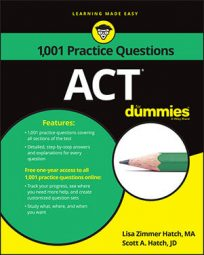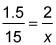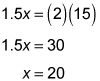##### ACTIf you want to aim for a ratio of 60 right answers to zero wrong answers on the ACT Math exam, then you should definitely brush up on your ratio skills.

In the following practice questions, you'll use ratios to determine the possible multiples of two numbers, and then to calculate the correct proportions of a bedroom in an architectural blueprint.

## Practice questions

1. After a hockey game, Bernie checks his body and finds that he has three bruises for every five cuts. Which of the following could be the total number of bruises and cuts on Bernie's body?

A. 53 B. 45 C. 35 D. 33 E. 32

2. A blueprint is to be an exact plan of a house's main bedroom on a reduced scale. If the main bedroom on the blueprint measures 18 inches long by 24 inches wide, the homeowner's bedroom of 15 feet long is how many feet wide?

A. 24 B. 22 C. 20 D. 12 E. 10

1. The correct answer is Choice (E).

Add the numbers in the ratio: 3 + 5 = 8. The total must be a multiple of 8 (or, looking at it another way, the total must be evenly divisible by 8). Only Choice (E) is a multiple of 8.

2. The correct answer is Choice (C).

You can eliminate Choices (D) and (E) because the width of the room on the plan is greater than its length and therefore the length of the actual room must be greater than its width of 15 feet. To find the ratio of the plan to the actual bedroom, set up a proportion. The ratio of the plan's bedroom length to the actual bedroom length is 18 inches to 15 feet. The answer has to be in feet, so change inches to feet. The 18 inches is the same as 1.5 feet and the 24 inches is the same as 2 feet, so the ratio of the plan's width to the room's width in feet is

and the proportion to find the width isCross-multiply and solve for x:The homeowner's bedroom measures 20 feet wide.